# Interval

(diff) ← Older revision | Latest revision (diff) | Newer revision → (diff)

A space-time interval is a quantity characterizing the relation between two events separated by a spatial distance and a time duration. In special relativity theory the square of an interval is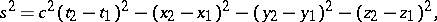whereis the velocity of light,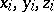are the space coordinates and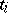are the corresponding points in time (for more details, see Minkowski space).

In general relativity theory one considers the interval between two infinitesimally-close events:where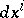is the infinitesimal difference of the space-time coordinates of these events and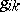is the metric tensor.

A space-time interval with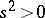is called a time-like space-time interval, and one with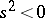is called a space-like space-time interval.